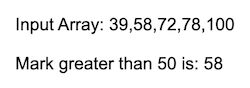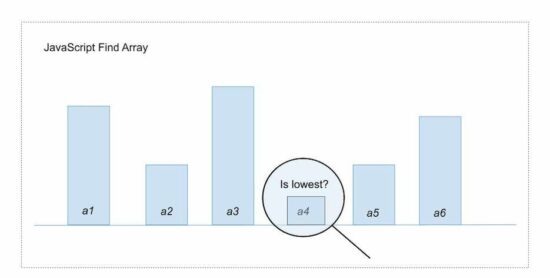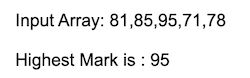# JavaScript Find to Get Array Element or Object

Learning arrays is one of the fundamental needs in learning programming. We have seen many articles previously to learn the power of arrays. This article is for learning how to find array elements in JavaScript.

The Javascript find method is to get an element or object from the input array. It receives a callback function to add the find conditions.

It gets the first element that satisfies the condition in the callback function. If no array elements satisfy the condition, then it returns ‘undefined’.

Look at the below quick example to see how the JavaScript find() is working. It gets the first occurrence of a mark that is greater than 50 from an array of marks given.

## Quick Example

examples/quick-example.htm

``````<script>
const mark = [ 39, 58, 72, 78, 100];
function checkmark(mark){
return (mark > 50);
}

var findResult  = mark.find(checkmark);
document.write("Input Array: " + mark +"<br/><br/>");
document.write("Mark greater than 50 is: " + findResult);
</script>
``````

It prints the following output in the document window.## JavaScript find syntax and parameters

Let’s know the syntax of find() to search elements from a collection of JavaScript array elements.

The JavaScript find() function can be used with different syntax. Below code snippets show the valid syntaxes of this function.

This function accepts the callback function to apply the search condition. It has the ‘this’ parameter which supplies the object and changes the default context. It is an optional parameter.

It supports the arrow function to map the array components to the callback function. We have used the arrow function and this parameter in JavaScript forEach() examples.

``````// JavaScript find() with external callback
find(callback, this)
``````
``````// Define inline callback function
find(function callback(element, index, array) { ... }, this)
``````
``````// Arrow function
find((element, index, array) => { ... } )
``````

### Parameters

Javascript find() accepts two Parameters, the callback function and this parameter.

#### callback

It applies conditions on which the JavaScript find() picks elements from the array. It can be defined as an inline or an external function.

#### this Parameter

By default, JavaScript takes the window context. The this’ parameter changes the default context to the enclosed context. This is optional.

#### Parameters of JavaScript find() callback

The callback function accepts 3 parameters. Those are the input array-related references listed below.

• element
• index
• array

The index and array are optional parameters. The array parameter refers to the input array parsed by the JavaScript find() function.

Let us see some highlights on the JavaScript find() method. It is one of the widely used array functions to get the elements that meet the conditions applied.

• This method accepts and executes the callback for every element of the array. Once the callback returns the first match, then the find() will stop.
• It finds all the array elements even the unassigned array holes of a sparse array.
• The Javascript find() will not manipulate the input array. But the callback function can change the input array.
• It’s an alternate for the JavaScript ‘Array.filter’ only if it is enough to get the first match. We have created a custom function with JavaScirpt find(). It returns multiple matches like Array.filter().

## Related Functions

There are many functions available in JavaScript that helps to find array elements.

• findIndex():- It returns the index of the element that satisfies the condition in the callback.
• includes():- It checks if a value exists in an input array or not; unlike JavaScript find() it is not using a callback.
• some():- returns true if any one of the array elements satisfies the condition; Or returns false.

## More Examples

### Javascript find with a callback – Get the highest mark from an array

The below code applies JavaScript find to get the highest marks from an array. It processes a marks array given as an input.

It checks each element of the input array. Then, it updates the highestMark if the condition satisfies. Then, it prints the highest mark to the browser.

examples/find-with-callback.htm

``````<script>
const mark = [ 81, 85, 95, 71, 78];

var highestMark = 0;
function findHighestMark(mark, index, array){
if(parseInt(mark) > parseInt(highestMark)) {
highestMark = mark;
}
}
mark.find(findHighestMark);

document.write("Input Array: " + mark +"<br/><br/>");
document.write("Highest Mark is : " + highestMark);
</script>
``````### How to use the JavaScript find() with this parameter

The ‘this’ parameter is used to pass an object to replace the default \$(window) context. This example passes a value to check if it exists on the given input array.

It applies the condition in the inline callback of the JavaScript find() function.  This code creates a JavaScript class and defines its properties and methods.

The filter() method executes JavaScript find() to check if an element exists. This method returns a boolean true or false based on the array element existence.

examples/find-with-this-parameter.htm

``````<script>
class ColorsList {
constructor(items) {
this.colorsArray = ["Red", "Green", "Blue"];
}

filter = function(entry) {
var output = false;
this.colorsArray.find(function countEntry(element,index,array) {
if(element == entry) {
output = true;
}
}, this);
return output;
}
}

const obj = new ColorsList()
var response = obj.filter("Yellow");
if (response==true){
document.write('Exist');
} else {
document.write('Not exist');
}
</script>
``````

### How to use arrow function in JavaScript find()

This example uses the JavaScript arrow function. It applies the condition on the array elements.

The Javascript find() function checks if the product_name property is equal to ‘Computer’. It returns the array element satisfying this condition.

This program uses an array of objects in an input array. It prints the product properties of the element satisfying the find() condition.

examples/find-with-arrow-function.htm

``````<script>
const productdetails = [
{product_name: 'Computer', stock: "200"},
{product_name: 'Phone', stock: "150"},
{product_name: 'Bike', stock: "100"}
];

const result = productdetails.find( ({ product_name })  => product_name === 'Computer');
document.write("Product_Name: "+result.product_name+"<br><br>");
document.write("Stock: "+result.stock);
</script>
``````

### Find all elements satisfying condition in the callback

This example uses JavaScript find() and defines a custom function. This function checks the conditions and gets all the elements instead of the first one.

This custom function simulates the behavior of the Array.filter() function.

examples/javascript-find-all.htm

``````<script>
const marks = [ 90, 48, 92, 95, 60];
var output = [];
marks.find(checkmarks);
function checkmarks(element, index, array) {
if(element >= 90) {
output.push(element);
}
}
document.write("Input Array: " + marks +"<br/><br/>");
document.write("All Marks >= 90 are : " + output);
</script>
``````

### JavaScript find index

Like the JavaScript find(), the findIndex() processes the array until it finds the match. The difference is findIndex() returns array index instead of the value.

This example finds the array element greater than 80. It applies a condition on the callback that returns the array element index.

examples/javascript-find-index.htm

``````<script>
const inputArray = [15, 24, 88, 10, 12];

function isLargeNumbers(element){
return (element > 80);
}

var index = inputArray.findIndex(isLargeNumbers)
document.write("Array Index: "+index);
</script>
``````

### JavaScript find() on sparse array

In the JavaScript find() method’s highlight, we have seen that it also processes array holes.

This example script inputs a sparse array to check this behavior of the find() method.

The callback processes the unassigned elements of the array. The inline callback returns undefined if it found an array hole.

examples/javascript-find-on-sparse-array.htm

``````<script>
const inputArray = [15, 24, , , 12];

function isLargeNumbers(element){
return (element > 80);
}

document.write("Input Array: [" + inputArray +"]<br/><br/>");
var index = inputArray.find(function (element, index){
if(element != undefined) {
document.write("Value: "+element+" Position: "+index+"<br/><br/>");
} else {
document.write("<b>No value at position "+index+"</b><br/><br/>");
}

});
</script>
``````

## Conclusion

Thus, we have seen how to use JavaScript find() method to search an array. We saw various examples in JavaScript to apply the find() condition on the input array.

The quick example gives a basic code to learn how the JavaScript find() function works. The other examples demonstrate the usage of this function. These examples vary in the use of possible parameters and specifications.

We have also seen some related JavaScript functions like filter(), findIndex() and more.